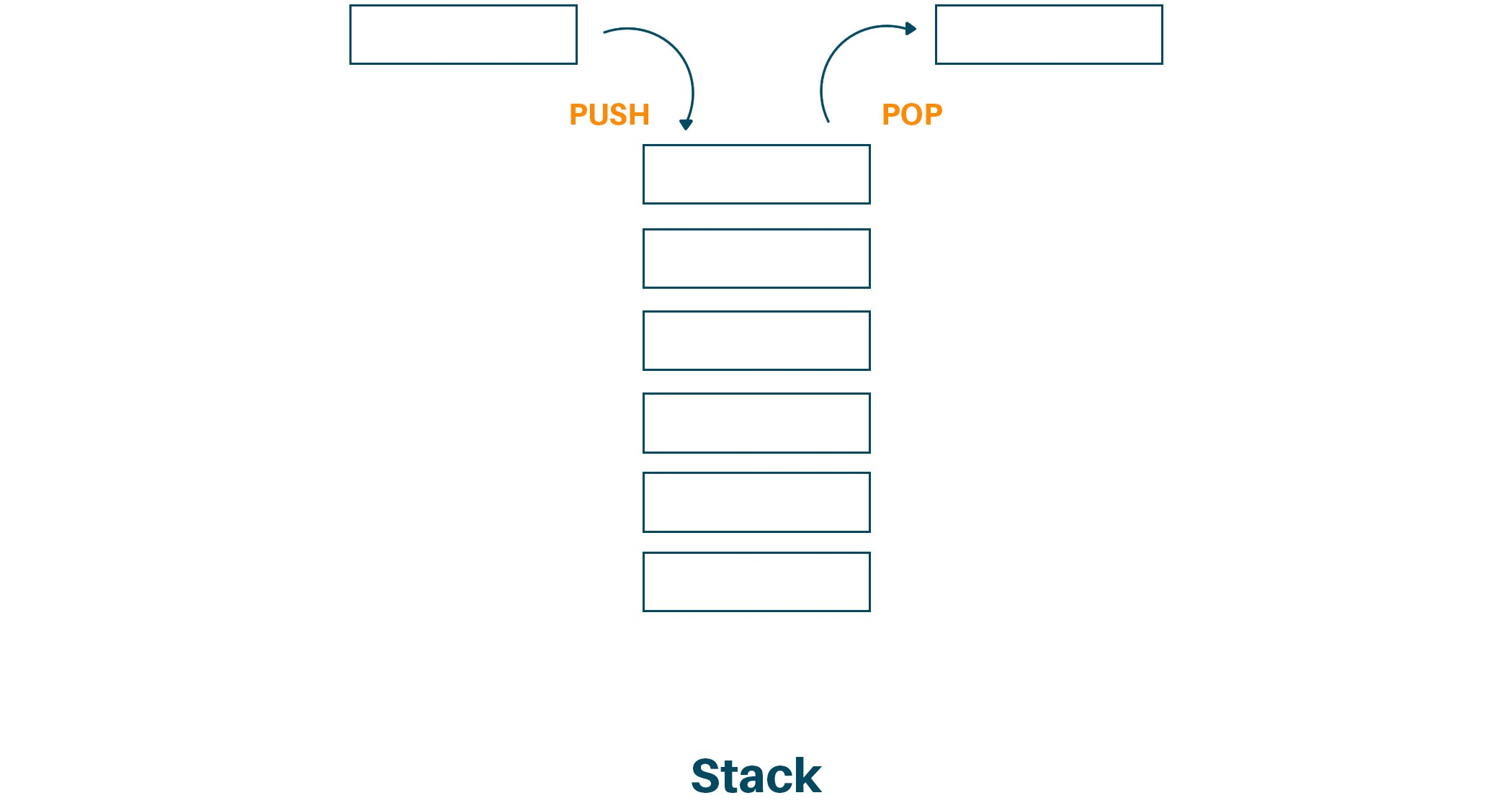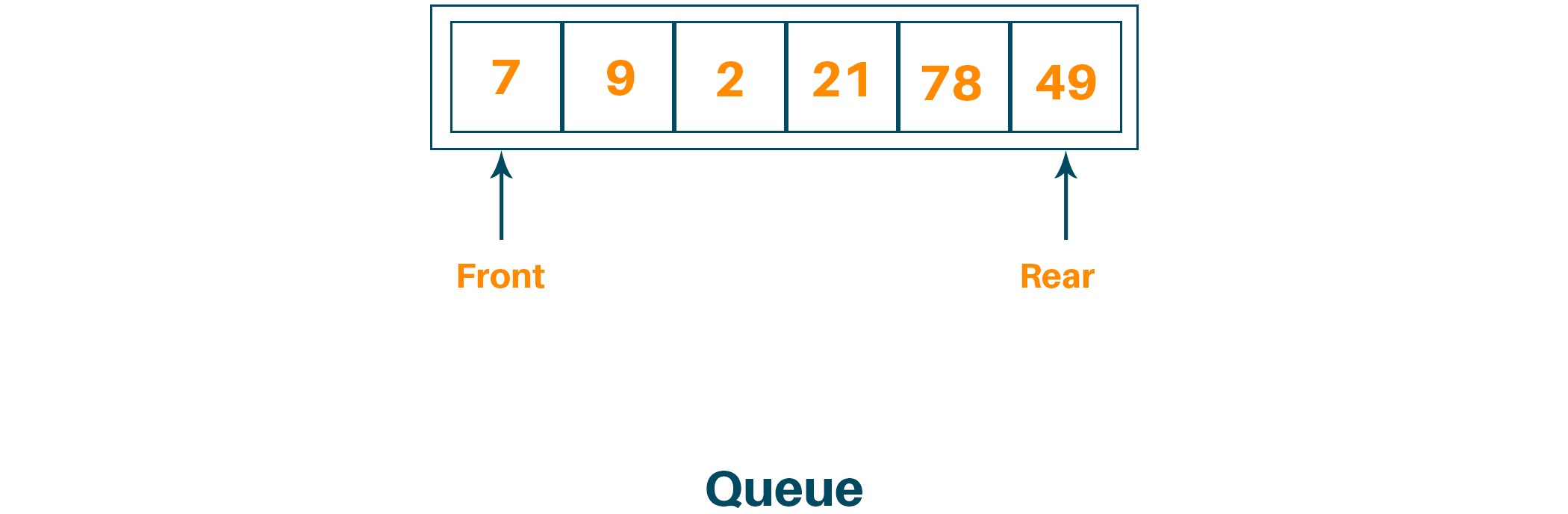# Stack vs Queue | 12 Difference Between Stack and Queue

In this article, we will study stack vs queue in detail along with the comparison chart between stack and queue data structure. At last, we will understand the practical application of stack and queue individually. So, let's get started!

## What is Stack?

Stack is a linear data structure that follows the specific order to perform the operations. For example, if we want to access the element in the array we can do it any time but in the case of stack data structure, there is only one sequence to access the element. In the stack, we insert the element from one end with push operation and delete the element from the same end using pop operation. The end of the stack used to perform all the operations is called the top of the stack. Therefore, a stack follows the LIFO (Last In First Out) principle, which means the element that is inserted last will be the first element to come out of the stack. The most important thing to remember in the stack data structure is that it stores the elements of the same data type only.Let us understand the condition to check whether the stack is empty for full:

### Condition to Check if Stack is Empty

```int Empty()
{
if(top==-1)
return 1;
else return 0;
}
```

### Condition to Check if Stack is Full

```int Full()
{
if(top==MAX-1)
return 1;
else
return 0;
}
```

## What is Queue?

The queue is a linear data structure in which we can insert the element from one side of the list and delete the element from the other side of the list. The end of the list from where the elements are inserted is called the rear end and the end from where the elements are deleted is called the front end. Therefore, the queue data structure follows the FIFO(First In First Out) principle, which means the element inserted first from the rear end will be the first element to be deleted from the front end. The insertion technique in the queue data structure is called enqueue operation and the deletion technique in the queue data structure is called dequeue operation. While performing operations in the queue, there are two pointers, front pointer and rear pointer, where the front pointer is used to point the element that is added first in the queue and the rear pointer is used to point the element which is added last in the queue. Also, just like stack data structure, we can only store elements of the same data type in the queue data structure.Let us understand the condition to check if the queue is empty or full

### Condition to Check if Queue is Empty

```int Empty()
{
if(front==-1 || front==rear+1)
return 1;
else
return 0;
}
```

### Condition to Check if Queue is Full

```int Full()
{
if(rear==MAX-1)
return 1;
else
return 0;
}
```

## Difference between Stack and Queue

### Queue

The stack is based on LIFO(Last In First Out) principle The queue is based on FIFO(First In First Out) principle.
Insertion Operation is called Push Operation Insertion Operation is called Enqueue Operation
Deletion Operation is called Pop Operation Deletion Operation is called Dequeue Operation
Push and Pop Operation takes place from one end of the stack

Enqueue and Dequeue Operation takes place from a different end of the queue

The most accessible element is called Top and the least accessible is called the Bottom of the stack

The insertion end is called Rear End and the deletion end is called the Front End.

Simple Implementation  Complex implementation in comparison to stack
Only one pointer is used for performing operations  Two pointers are used to perform operations

Empty condition is checked using

Top==-1

Empty condition is checked using

Front==-1||Front==Rear+1

Full condition is checked using

Top==Max-1

Full condition is checked using

Rear==Max-1

There are no variants available for stack

There are three types of variants i.e circular queue, double-ended queue and priority queue

Can be considered as a vertical collection visual

Can be considered as a horizontal collection  visual

Used to solve the recursive type problems

Used to solve the problem having sequential processing

## Application of Stack Data Structure

There are many applications of stack data structure in real life. Some of them are as given below:

1. Stack is used for expression evaluation
2. Stack is used for parenthesis matching while working with expressions.
3. Stack is used in expression conversion. For example, infix to postfix or prefix to postfix
4. Stack is used in memory management
5. It is used in java virtual machine
6. It is used for the Backtracking problem-solving method
7. It is used in string parsing or string reversal.
8. Stack is used to matching the HTML tags in web development
9. Stack is also used in function call for recursive functions.

## Application of Queue Data Structure

There are many applications of queue data structure in real life. Some of them are as given below:

1. The queue is used as a waiting list when the resource is to be shared with multiple systems. For example, CPU scheduling or disk scheduling
2. It is used in the operating system for FCFS scheduling, semaphores, buffer for devices and spooling the printers
3. Queues are used in routers and switches
4. In networking, the queue is used when data is transferred asynchronously
5. Used in maintaining the playlist in media players
6. Used to handle interrupts in the operating system
7. The queue is used in the round-robin scheduling algorithm

## Conclusion

Therefore, in the above article we studied the difference between stack and queue as a data structure. As we saw stack and queue both are non-primitive, linear data structures with so many differences in certain ways like mechanism, structure, implementation and variants. But even though being different from one another, they have a lot in common like storing the same data type elements and with so many practical applications in real life.

### FavTutor - 24x7 Live Coding Help from Expert Tutors!